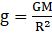Question 7. Explain why the value of g is zero at the centre of the earth.

Question 7.

Explain why the value of g is zero at the centre of the earth.

The value of ‘g’ changes as we go inside the earth. According to formula of. As we go deep inside the earth the Value of ’R’ decreases therefore according to formula the value of ‘g’ should increase. But it is not so because as we go inside the earth towards its center the part of the earth(M) responsible for the gravitational force also decreases that is the value of the ‘M’ also decreases.